# AP Computer Science A : Arrays

## Example Questions

### Example Question #1 : Arrays

Consider the following code:

int[] vals = {6,1,41,5,1};

int[][] newVals = new int[vals.length][];

for(int i = 0; i < vals.length; i++) {

newVals[i] = new int[vals[i]];

for(int j = 0; j < vals[i];j++) {

newVals[i][j] = vals[i] * (j+1);

}

}

What does the code above do?

The code creates a 2D array that contains the multiples for the members of vals, using each of those values to determine the number of multiples to be computed.

It performs a matrix multiplication on vals and newVals, storing the final result in newVals.

It sums the values in vals, placing the outcome in newVals.

It creates a 2D matrix with the vals array for every row.

The code creates a 2D array that contains all multiples from 1 to 5 for the members of vals.

The code creates a 2D array that contains the multiples for the members of vals, using each of those values to determine the number of multiples to be computed.

Explanation:

Let's look at the main loop in this program:

for(int i = 0; i < vals.length; i++) {

newVals[i] = new int[vals[i]];

for(int j = 0; j < vals[i];j++) {

newVals[i][j] = vals[i] * (j+1);

}

}

The first loop clearly runs through the vals array for the number of items in that array.  After this, it creates in the newVals 2D array a second dimension that is as long as the value in the input array vals.  Thus, for 41, it creates a 3rd row in newVals that is 41 columns wide.

Then, we go to the second loop.  This one iterates from 0 to the value stored in the current location in vals.  It places in the given 2D array location the multiple of the value.  Think of j + 1.  This will go from 1 to 41 for the case of 41 (and likewise for all the values).  Thus, you create a 2D array with all the multiples of the initial vals array.

### Example Question #2 : Arrays

Consider the following code:

public static void main(String[] args) {

int[] vec = {8,-2,4,5,-8};

foo(vec);

}

private static void foo(int[] x) {

for(int i = 0; i < x.length; i++) {

int y = Math.abs(x[i]);

for(int j = 0; j < y; j++) {

System.out.print(x[i] + " ");

}

System.out.println();

}

}

Which of the following represents a possible output for the program above?

8 8 8 8 8 8 8 8

-2 -2

4 4 4 4

5 5 5 5 5

-8 -8 -8 -8 -8 -8 -8 -8

8 8 8 8 8 8 8 8

4 4 4 4

5 5 5 5 5

37

64

4

16

25

64

64

-4

16

25

-64

8 8 8 8 8 8 8 8

-2 -2

4 4 4 4

5 5 5 5 5

-8 -8 -8 -8 -8 -8 -8 -8

Explanation:

In this code's loop, notice that it takes the absolute value of each element.  This is done on the line:

int y = Math.abs(x[i]);

This value is then used for the second loop, which goes for y times, each time outputting the value of the given member of the original array—but now with its particular sign value.  Thus, even numbers likewill be output multiple times (i.e. 8).  This is done line by line for each member of the parameter array.

### Example Question #3 : Arrays

Consider the following code:

public static void main(String[] args) {

double[][] matrix = {{1,6,7},{1,4,5}};

graphics(matrix);

}

private static double graphics(double[][] x) {

double r = 0;

for(int i = 0; i < x.length; i++) {

for(int j = 0; j < x[i].length; j++) {

r += x[i][j] * (i + 1);

}

}

return r;

}

What is the return value for graphics in the code below:

double[][] matrix = {{1,6,7},{1,4,5}};

graphics(matrix);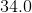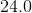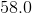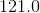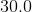Explanation:

The graphics method takes the 2D array matrix and then runs through each element.  This is the point of the double for loop in the method itself.  Notice that for each element it does several things.  First of all, it is clearly accumulating a value into the variable r.  Now, for each element, you are adding:

x[i][j] (the current value in the 2D array iteration)

TIMES

(i + 1), or, the current row number.  Thus, for the data given you are doing the following:

1* 1 + 1 * 6 + 1 * 7 + 2 * 1 + 2 * 4 + 2 * 5 = 34

### Example Question #4 : Arrays

For the following question, consider the following code:

public static void main(String[] args)

{

double[] vec = {4,8,10,18};

System.out.println(fun(vec));

privatestaticdouble fun(double[] x)

{

double p = 0;

for(int i = x.length-1; i > -1; i--)

{

p += x[i];

}

return p / x.length;

}

Which of the following is a possible output for this program?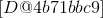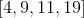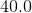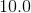Explanation:

First of all, you can eliminate the following two options very quickly, as the fun method returns a double value:

[D@4b71bbc9]

[4,9,11,19]

These are trying to trick you into thinking that it returns an array.  (The first value is really what would be the output, since there is no toString defined for the double array by default.)

Now, in fun, the loop simply runs through the values in the array, summing those up.  Notice, it runs "backwards" through the array, from the end to the beginning.  It then divides by the length of the array, giving you the average of the values in the array.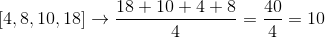### Example Question #5 : Arrays

Consider the following code:

String s = "I read logic for fun!";

boolean[] b = {true,false,true,false,false,true,true,false,true,false,true,false,false,true,false,true,true,false,true,false,false};

for(int i = 0; i < s.length(); i++)        {

char c = s.charAt(i);

if(!b[i]) {

c = Character.toUpperCase(c);

} else {

c = Character.toLowerCase(c);

}

System.out.print(c);

}

What is the output for this function code?

The code will throw an exception.

Explanation:

This code is using what are called parallel arrays.  The boolean array has one value for each character in the String s.  Notice the logic in the loop.  There is a condition:

if(!b[i]) {

c = Character.toUpperCase(c);

} else {

c = Character.toLowerCase(c);

}

This means that if the boolean is false, then you will make the given letter upper case.  (This is because of the ! in the condition. Be careful!)  Otherwise, you make it lower case.

Carefully walking through the code, you will get:

### Example Question #34 : Common Data Structures

Suppose your friend has the following lines of code that intend to find the first index of the first positive integer in array ... array[N-1], where array is an array of N integers

int i = 0;

while (array[i] >=0)

{

i++;

}

location = i;

Will your friend's code work as intended?

It never works.

It will work, but unexpectedly fail on occasion.

When array contains at least one negative integer

It works when there are no negative integers.

Yes, it works as intended.